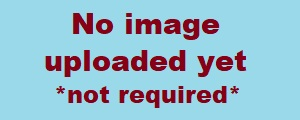Email : ren@techsolus.co.uk

Home

# C# Int.TryParse

The Code

```            Int32 MyNumber = 1;             if (int.TryParse(MyStringNumberToTest, out MyNumber) ==  false)             {                 MyNumber = 1;             }```

The Explanation

We need to check if an input, a string, is a number for whatever reason.

`Int32 MyNumber = 1;`

So we create an integer! You don't HAVE to give it a value but I find it helps.

`int.TryParse(MyStringNumberToTest, out MyNumber)`

What we are saying here is... as an integer we will TRY to PARSE this thing, this string, into a number. If this works we shall OUT that parsed number into MyNumber ready to use.

```            if (int.TryParse(MyStringNumberToTest, out MyNumber) ==  false)             {                 MyNumber = 1;             }```

Here we try the parse. If it works it returns true and we don't do any more as the parsed number is comfortably sat in MyNumber. But!! IF the parse fails because it's not a number then we set, default the number to 1.

Name

Comment